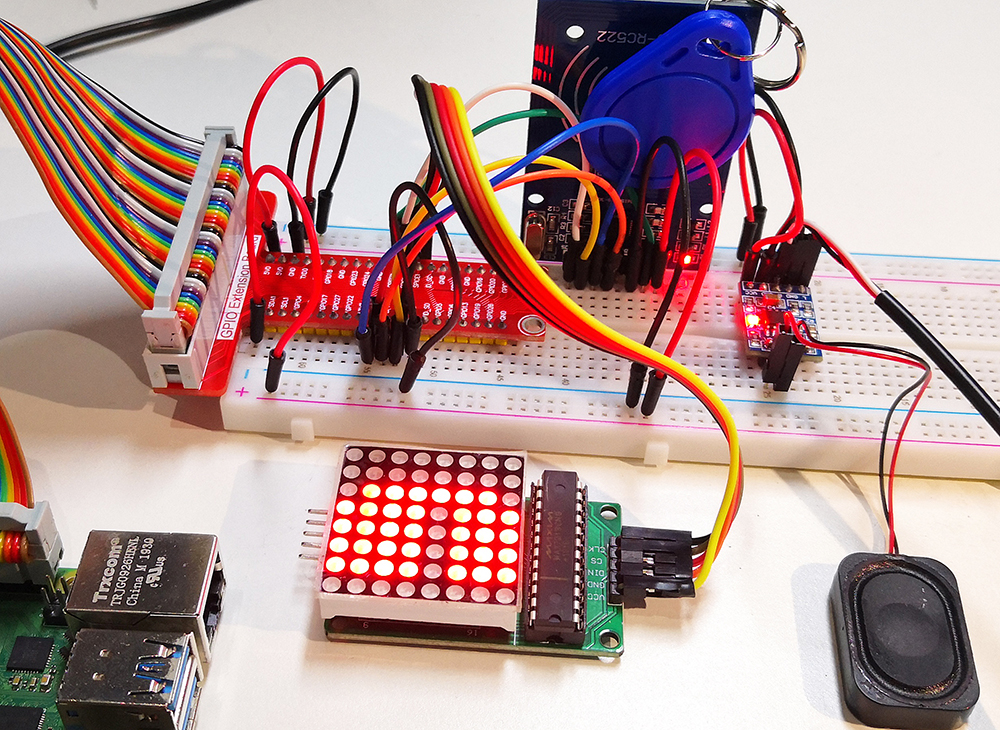# 4.1.19 AttendanceSystem¶

## Introduction¶

Let’s make a simple attendance system. When we scan the card, the Raspberry Pi will record our information and generate a csv file.

## Required Components¶

In this project, we need the following components.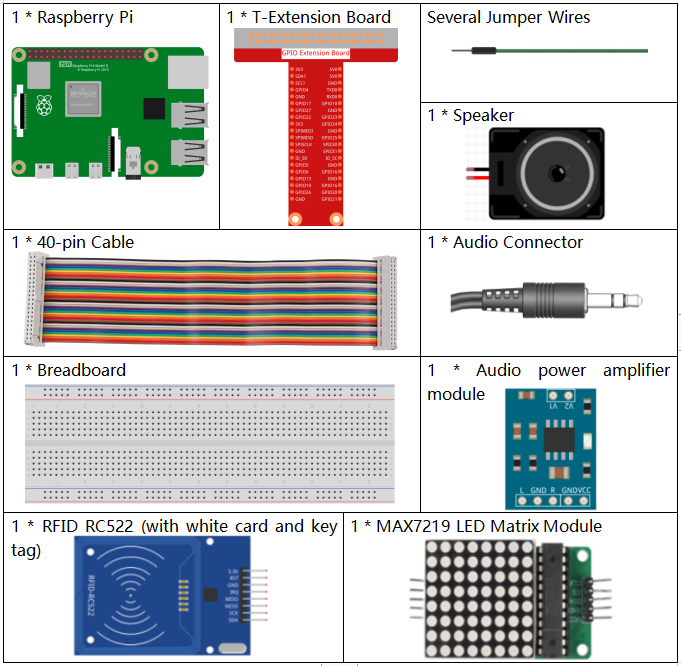Name

ITEMS IN THIS KIT

Raphael Kit

337

Raphael Kit

COMPONENT INTRODUCTION

GPIO Extension Board

Jumper Wires

Audio Module and Speaker

-

LED Matrix Module

MFRC522 Module

## Schematic Diagram¶

 T-Board Name physical wiringPi BCM GPIO25 Pin 22 6 25 SPIMOSI Pin 19 12 MOSI SPIMISO Pin 19 12 MISO SPICE0 pin 24 10 CE0 SPICE1 pin 26 11 CE1 SPISCLK Pin 23 14 SCLK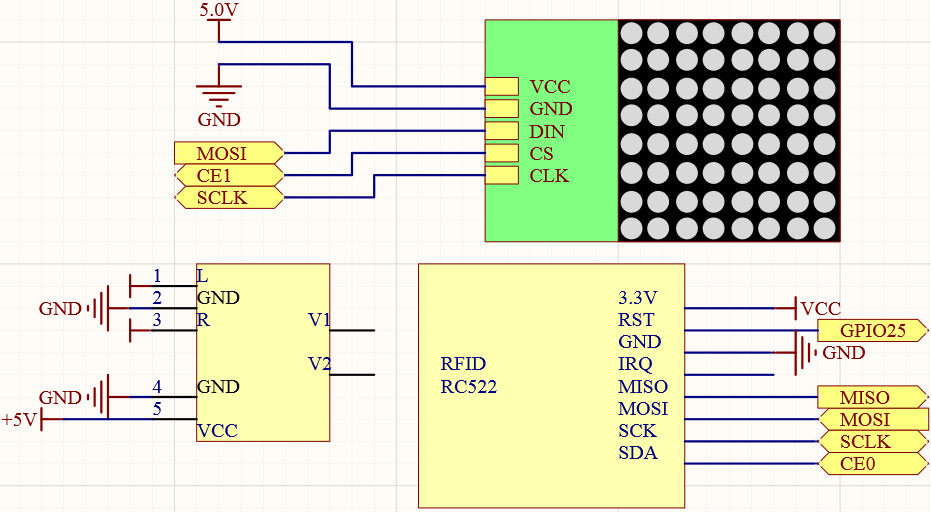## Experimental Procedures¶

Note

Turn on the SPI before starting the experiment, refer to SPI Configuration for details.

The Luma.LED_Matrix and the Spidev and MFRC522 libraries are also needed.

Step 1: Build the circuit.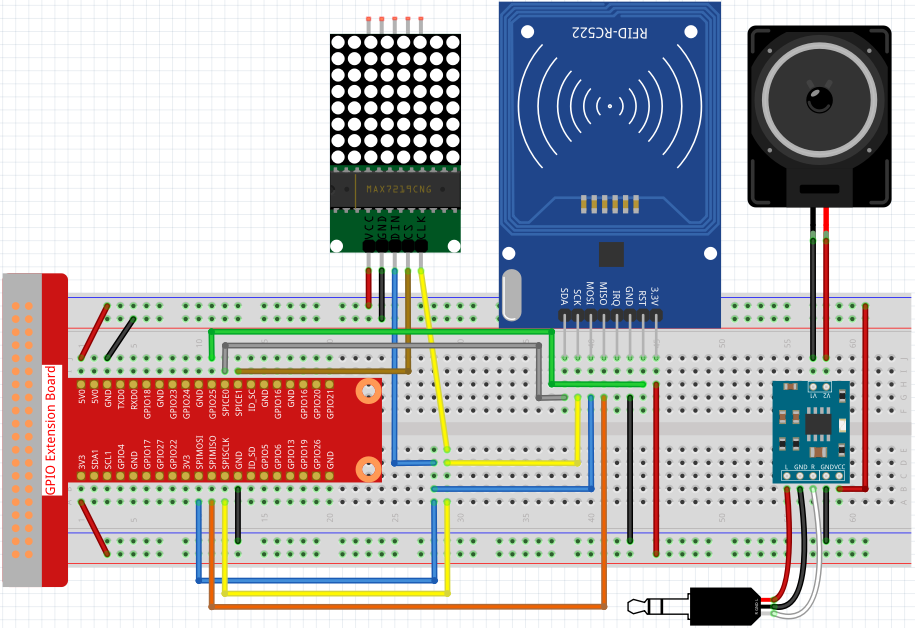Step 2: Run the `2.2.10_write.py` file to modify the content of the rfid card.

```cd ~/raphael-kit/python
sudo python3 2.2.10_write.py
```

Step 3: Enter the name (here we use `John``as an example) and press ``Enter` to confirm, then put the card on the MFRC522 module, wait for “Data writing is complete” to appear and take the card away, or rewrite the message to another card and exit by `Ctrl+C`.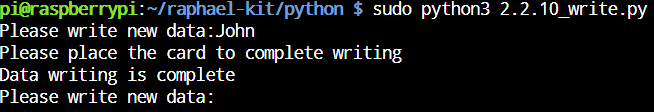Step 4: Get into the folder of code and run.

```cd ~/raphael-kit/python
sudo python3 4.1.19_Attendance_Machine.py
```

After starting the program, we put the RFID card close to the MFRC522 RFID Module, the Raspberry Pi will send out a voice to greet you and display it on the LED matrix.

We can also find a `.csv` file that records the time and list in the same directory. Open it with the nano command and you will see the record just now.

```sudo nano attendance_sheet.2021.06.29.csv
```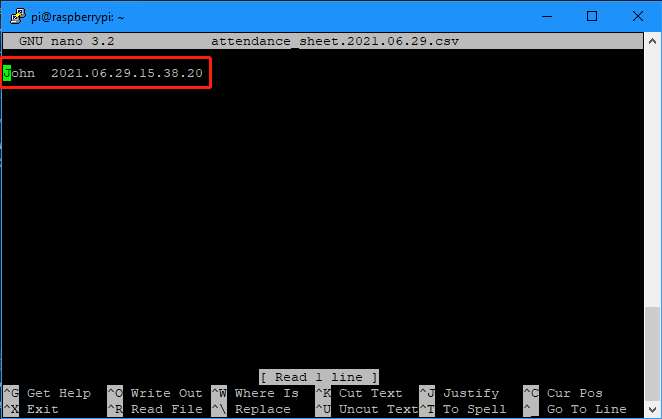Code

Note

You can Modify/Reset/Copy/Run/Stop the code below. But before that, you need to go to source code path like `raphael-kit/python`. After modifying the code, you can run it directly to see the effect.

```import time
from tts import TTS
import RPi.GPIO as GPIO
from mfrc522 import SimpleMFRC522
from luma.core.interface.serial import spi, noop
from luma.core.render import canvas
from luma.core.virtual import viewport
from luma.led_matrix.device import max7219
from luma.core.legacy import text
from luma.core.legacy.font import proportional, CP437_FONT, LCD_FONT

serial = spi(port=0, device=1, gpio=noop())
device = max7219(serial, rotate=1)
virtual = viewport(device, width=200, height=400)

tts = TTS(engine="espeak")
tts.lang('en-US')

attendance_statistics = {}

def get_time():
time.time()
year = str(time.strftime('%Y',time.localtime(time.time())))
month = str(time.strftime('%m',time.localtime(time.time())))
day = str(time.strftime('%d',time.localtime(time.time())))
hour = str(time.strftime('%H',time.localtime(time.time())))
minute = str(time.strftime('%M',time.localtime(time.time())))
second = str(time.strftime('%S',time.localtime(time.time())))
present_time = year + '.' + month + '.' + day + '.' + hour + '.' + minute + '.' + second
present_date = year + '.' + month + '.' + day
return present_date, present_time

def main():
while True:
print(id,name)
greeting = name.rstrip() + ", Welcome!"
present_date, present_time = get_time()
attendance_statistics[name.rstrip()] = present_time
tts.say(greeting)
with open('attendance_sheet.' + present_date + '.csv', 'w') as f:
[f.write('{0}  {1}\n'.format(key, value)) for key, value in attendance_statistics.items()]
with canvas(virtual) as draw:
text(draw, (0, 0), greeting, fill="white", font=proportional(CP437_FONT))
for offset in range(95):
virtual.set_position((offset,0))
time.sleep(0.1)

def destroy():
GPIO.cleanup()
pass

if __name__ == '__main__':
try:
main()
except KeyboardInterrupt:
destroy()
```

## Code Explanation¶

In order to better understand the program, you may need to complete 1.1.6 LED Dot Matrix , 2.2.10 MFRC522 RFID Module and 3.1.4 Text-to-speech first.

```def get_time():
time.time()
year = str(time.strftime('%Y',time.localtime(time.time())))
month = str(time.strftime('%m',time.localtime(time.time())))
day = str(time.strftime('%d',time.localtime(time.time())))
hour = str(time.strftime('%H',time.localtime(time.time())))
minute = str(time.strftime('%M',time.localtime(time.time())))
second = str(time.strftime('%S',time.localtime(time.time())))
present_time = year + '.' + month + '.' + day + '.' + hour + '.' + minute + '.' + second
present_date = year + '.' + month + '.' + day
return present_date, present_time
```

Use the `get_time()` function to get the current timestamp and return two values. Among them, `present_date` is accurate to the number of days of the current timestamp, and `present_time` is accurate to the number of seconds of the current timestamp.

```id, name = reader.read()
greeting = name.rstrip() + ", Welcome!"
present_date, present_time = get_time()
attendance_statistics[name.rstrip()] = present_time
```

The `reader.read()` function reads the name information, and then creates a greeting. Then an `attendance_statistics` dictionary is generated, and `name.rstrip()` and `present_time` are stored as keys and values.

```tts.say(greeting)
```

Say a greeting through the speaker.

```with open('attendance_sheet.' + present_date + '.csv', 'w') as f:
[f.write('{0}  {1}\n'.format(key, value)) for key, value in attendance_statistics.items()]
```

Write the `attendance_statistics` to the .csv file.

```with canvas(virtual) as draw:
text(draw, (0, 0), greeting, fill="white", font=proportional(CP437_FONT))
for offset in range(95):
virtual.set_position((offset,0))
time.sleep(0.1)
```

Scroll to display this greeting.

Phenomenon Picture